### Home > ACC7 > Chapter 8 Unit 9 > Lesson CC3: 8.3.1 > Problem8-130

8-130.

Find the value of each indicated angle in the following figures. Do not use a protractor. Use the properties of straight and vertical angles to help you.

1.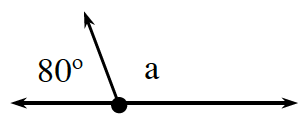These angles are supplementary. $180° - 80° = a$

1.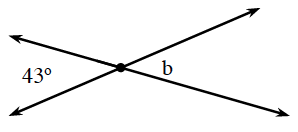$43°$ and b are vertical angles. What does that tell you about the measure of angle $b$?

1.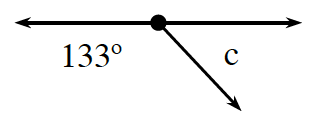Set up an equation relating $133°$ and $d$.
$133° + c = 180°$.

Subtract 133° from both sides.
$133° + c = 180$°.
$−133° −133°$
$c = 47°$

$47^◦$

1.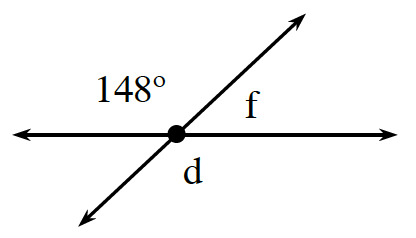d and $148°$ are vertical angles, and f and $148°$ are supplementary.

$d=148°,f=32°$

1.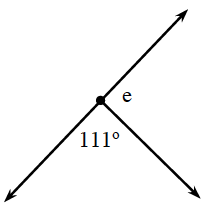$111° + e = 180°$. Refer to part (c) for the steps to solving this problem.